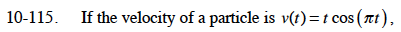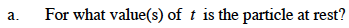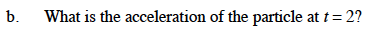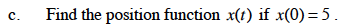### Home > CALC > Chapter Ch10 > Lesson 10.3.1 > Problem10-115

10-115.
1. If the velocity of a particle is v(t) = t cos (πt), Homework Help ✎

1. For what value(s) of t is the particle at rest?

2. What is the acceleration of the particle at t = 2?

3. Find the position function x(t) if x(0) = 5.When t = 0 or cos(πt) = 0.a(t) = cos(πt) – t(π)sin(πt)$s(t)=\int v(t)dt$

Use integration by parts. Let f = f and dg = cos(πt)dt.

Solve for C given x(0) = 5.